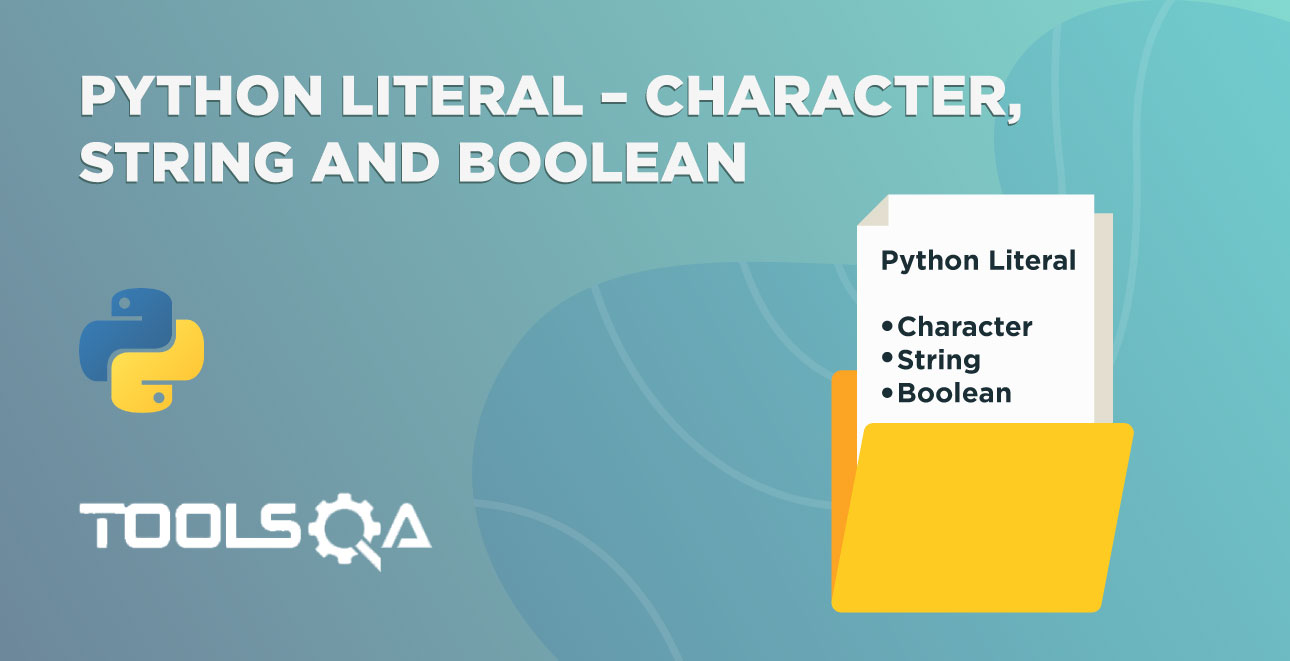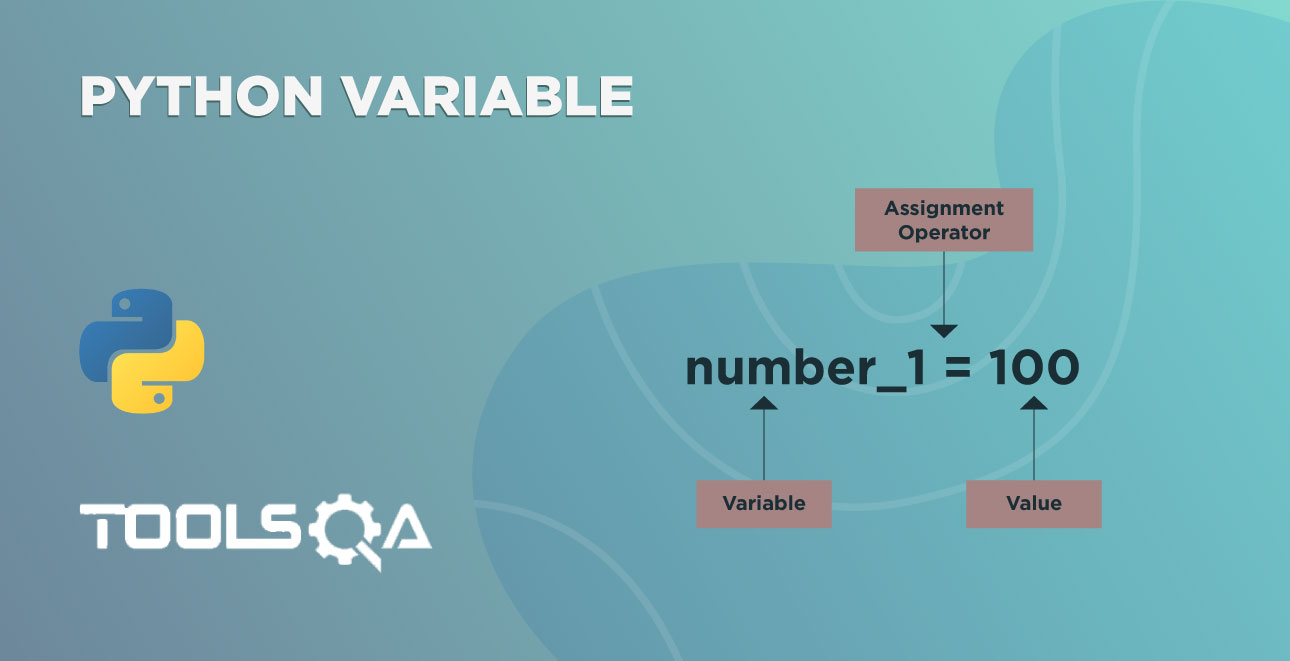We discussed Python Data Types and Python Literals in the last tutorial. So what do we do with these data types? We can perform operations on those data types using the operators. Consequently, in this tutorial, we are going to learn about Python Arithmetic Operators. We will discuss the below topics.

• Introduction
• Arithmetic Operators in Python
• Exponentiation
• Multiplication
• Division
• Floor/Integer Division
• Modulo
• Subtraction
• Unary Operators
• Precedence and Associativity
• Precedence
• Associativity Or Bindings
• Role of Parenthesis

## Introduction on Python Arithmetic Operators

Before diving into the tutorial, let's see a demo. Let's run the following code in IDE.

``````print(2 + 3)
``````

Consequently, you will get the output as 5. Which, in turn, is the sum of 2 and 3.

So far, we have seen print function displaying the literals that we are passing to it as arguments. In addition to that, the print function displays the result of the expression 2 + 3. Therefore, we can surely use this as a calculator! For example,

• print(2 + 5 - 3)
• print(7 / 4 * 3)

So far, so good? Let's discuss a few terminologies.

2 + 3 is an expression. Moreover, + is an operator, and 2, 3 are operands.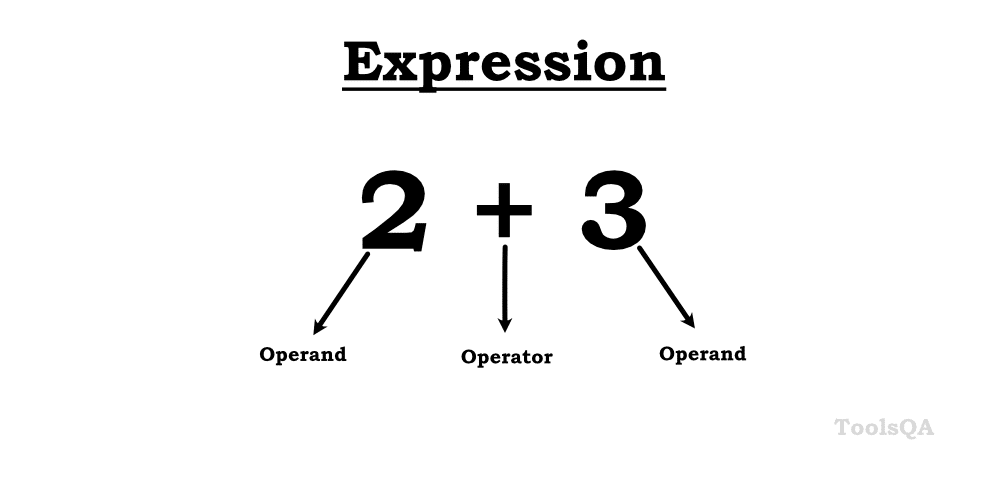The combination of operators and operands is known as an expression. Moreover, Literals form the most simplistic expression in programming.

An operator is a symbol that can perform some operation on the data. For example, + can give you the sum of two numbers. Additionally, Python has different types of operators. We are going to see the arithmetic operators as these are fundamental in any programming language.

In addition to the above, the data on which operators perform some operation is an operand.

## Python Arithmetic Operators

Python Arithmetic operators take numeric values as operands and return a single value as a result. Additionally, we have the following arithmetic operators in Python.

Symbol Operator
** Exponentiation
* Multiplication
/ Division
// Floor Or Integer Division
% Modulo
- Subtraction

Let's discuss these in detail.

### Exponentiation Operator ()**

The symbol, double-stars ()**, is an exponential operator in Python. Additionally, the left operand of the operator is base. Similarly, the right one is an exponent. Moreover, it calculates the value base to the power of exponent, i.e., baseexponent. For example, we will represent the 2 to the power of 5 as 25. Conclusively, we can calculate the value of 25 using the ** operator as 2 ** 5.

Try the following examples in IDE.

``````# exponential operator
print(2 ** 5)
print(2. ** 5)
print(2 ** 5.)
print(2. ** 5.)
``````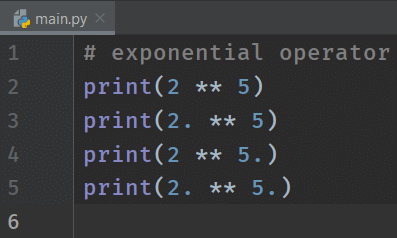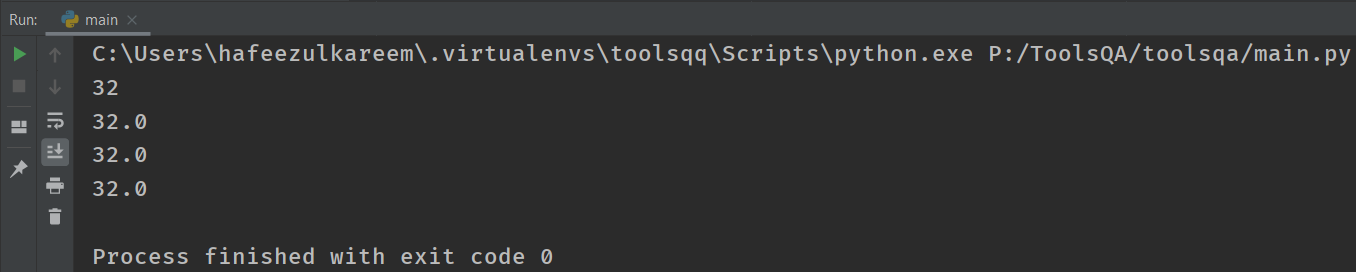Did you figure out that the first result is an integer while the last three are floating? Here is the logic behind it:

• Firstly, when all the operands of exponentiation are integers, then the result is an integer too.
• Secondly, when at least one operand is a float, then the result is a float.

### Multiplication Operator () in Python*

The multiplication operator multiplies any two numbers and gives the result. Additionally, we represent it using the asterisk ()* symbol in Python.

Let's run the below code: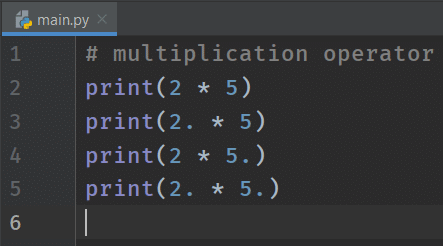As you can see, the multiplication operator also follows the same rules as exponentiation for calculating integer or float values.

### Division Operator (/) in Python

The division operator helps to divide a number with another number. Moreover, we can recognize the division operator using the symbol slash (/).

Try the below code on your own.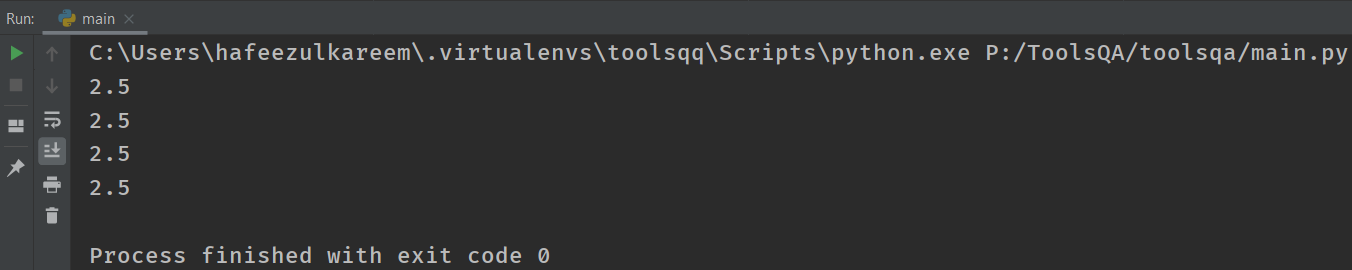The result is as expected.

Let's try one more. Try to print (4/2). What did you get? 2.0 !! Were you expecting 2?

The explanation for this is simple. The result of the division operator is always a float irrespective of the type of operands.

### Floor Or Integer Division (//) in Python

The double slash (//) is the symbol used to represent floor division (or Integer division). Moreover, it will round off the result to an integer value. For example,

``````# floor division operator
print(5 // 2)
print(5. // 2)
print(5 // 2.)
print(5. // 2.)
``````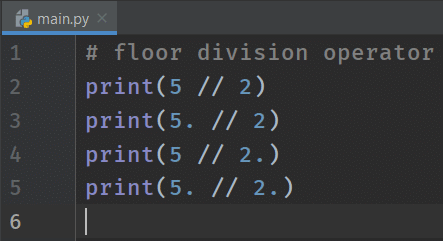If you see the output/ result of the first operation, i.e., integer by integer is an integer value. Similarly, the remaining results are floats. Moreover, it's on the same lines as exponentiation and multiplication.

Now let's understand why the rounding off happened to 2 and not 3?

The rounding off happens to the nearest integer value that is less than the actual result. In the above case, the nearest integer that is less than 1.5 is 1. For example,

print (11//3) = 3

print (5//5) = 1

Let's try some negative numbers now.

``````print(3 // -2)
print(-3. // 2)
``````

Consequently, the result of the above code is -2 and -2.0. Why?

The result of the code with / operator is -1.5 and -1.5.  The floor division (//)  rounds the result to the nearest and lesser integer value. That is to say, -2 is lesser than -1. Therefore, the output is -2 and -2.0.

### Modulo Operator (%) in Python

The percent (%) sign is the symbol to represent the modulo operator.

Additionally, it will give you the remainder left after performing the floor division.

The result of the expression 5 % 2 is 1. Let's run the following code and see it.

``````# modulo operator
print(5 % 2)
``````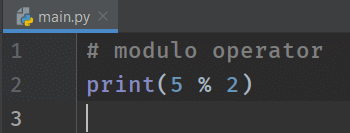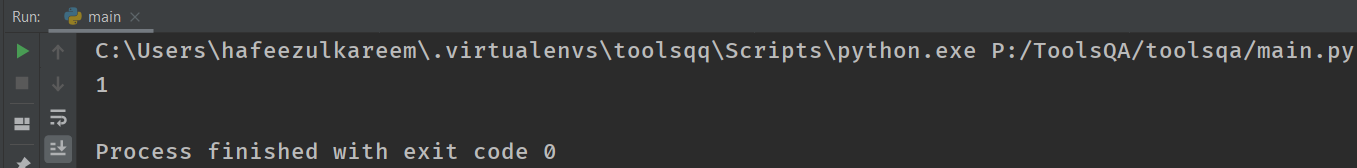The result is 1. But How? Let's see it in three steps.

1. First, perform the floor division, i.e., 5 // 2 = 2.
2. Second, multiply the above result and divisor, i.e., 2 * 2 = 4.
3. Finally, subtract the previous result from the dividend, i.e., 5 - 4 = 1.

The result you got in the last step is the final output of the expression 5 % 2. It might sound a bit tricky, but if you practice a bit, it's pretty easy!

Try the below code on your own.

``````print(5. % 2)
print(5 % 2.)
print(5. % 2.)
``````

Did you get  2, 2.0, and 2.0?

To conclude, you would have realized that the % operator also follows the same integer and float rules like multiplication and other operators.

### Addition Operator (+) in Python

We have to use the plus (+) sign for the addition operation. Moreover, it returns the sum of the operands. For example,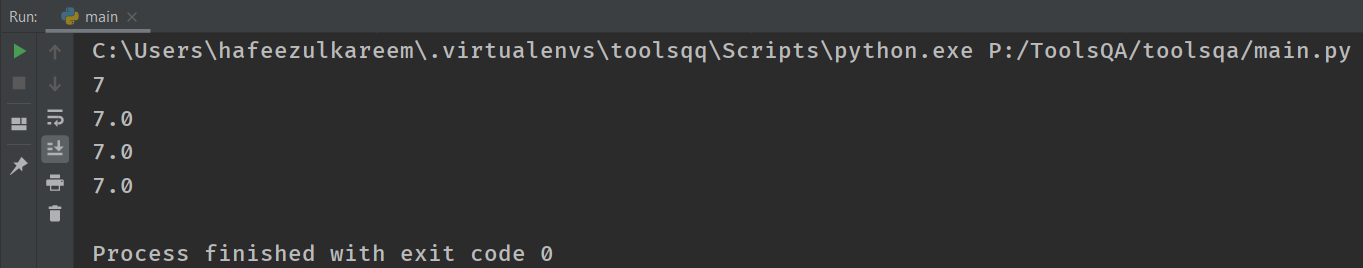### Subtraction Operator (-) in Python

Pretty much self-explanatory! We will use the same minus (-) that we use in the usual maths for subtraction. For example,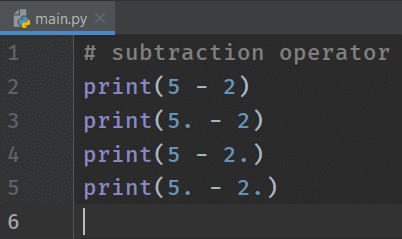The result is on expected lines. Not much to explain here!

### Unary Operators (+, -) in Python

The operators that we have learned so far are called binary operators. In other words, it means that we need two operands to carry out an operation.

Similarly, unary operators need only one operand to perform their respective operations.

We have already seen + and - as binary operators (addition and subtraction), Let's see how we can use them as Unary operators.

For example,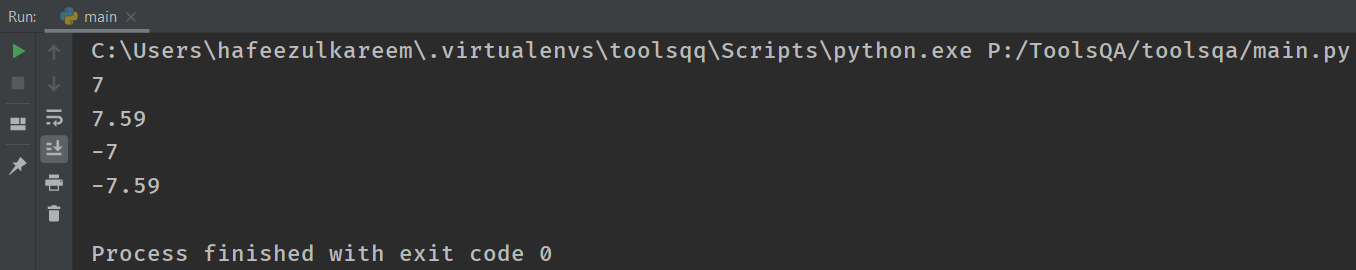It's not encouraged to write print(+7). Instead, we should be writing it as a print(7).

Let's try to mix the binary operator (+ and -) with the Unary operator (+ and -).

Try the below code on your own. The result should be self-explanatory!

print(3 + -3)

print(-3.5 + -2.5)

print(7 - -5)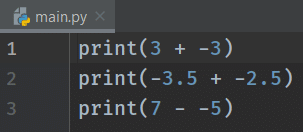So far, so good? It pretty much covers the Python arithmetic operators. Next, we will see a fundamental concept of Operator Precedence and Associativity.

## Precedence and Associativity

Let's understand it with a simple example.

Compute the expression 2 + 5 * 7

Based on which operator you evaluate first, you will either get 37 or 49 as an answer. So let's understand some rules around it.

### Precedence

The correct answer for the expression 2 + 5 * 7 is 37. We got the result by performing multiplication first, and then the addition operation. The expression evaluates as 2 + 5 * 7 => 2 + 35 => 37.

Run the same example in IDE and check the answer.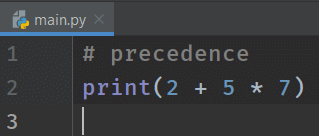So why is multiplication evaluated before addition?

It's because Python has already defined the precedence of the operators, based on which the expression evaluation happens. This precedence is the same across all the other languages as well.

Let's look at the precedence table, which defines in which order the operators evaluate.

#### Precedence Table

Precedence Operators
1 +, - (unary)
2 **
3 *, /, %, //
4 +, - (Binary)

The operators with number 1 have the highest precedence, and the operators with number 4 have the lowest precedence. Multiple operators in a row mean that they all have the same precedence.

For example,

print( 75 / 2)*

Based on what we have learned so far, both * and / have the same precedence. So how do we evaluate this one?  We need to learn another concept called associativity to get to this answer.

### Associativity Or Binding

Associativity (also called as bindings) defines the order of evaluation performed by the equal precedence operators. There are two types of bindings, i.e., left and right binding.

Most of the Python's operators have left associativity. Which, in turn, means the evaluation of the expression happens from left to right. Some operators have the right associativity, and the flow of execution is from right to left. For example,

``````# associativity
print(7 - 4 - 1)
``````

We can perform the above expression from left to right or right to left. Look at the result from both directions.

• left to right: 7 - 4 - 1 => 3 - 1 => 2
• right to left: 7 - 4 - 1 => 7 - 3 => 4

We got two different results. Run the example in IDE to find out the associativity of the - operator.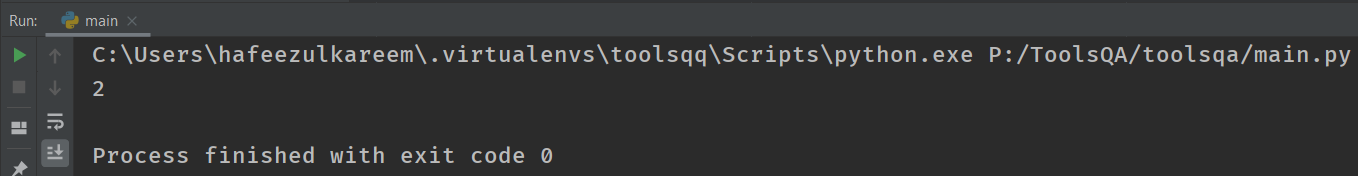We got the result as 2. So, that shows that the associativity of - is left.

Let's take an example to see the right associativity operator from the list of operators we have discussed.

``````# associativity
print(5 ** 1 ** 2)
``````
• left to right: 5 ** 1 ** 2 => 5 ** 2 => 25
• right to left: 5 ** 1 ** 2 => 5 ** 1 => 5

Evaluate the expression in Python IDE and compare it.The answer is 5. Hence the associativity of the exponential operator is right.

Let's have a look at the associativity of the operators we have learned so far.

Operators Associativity
+, - (unary) left to right
** right to left
*, /, %, // left to right
+, - (Binary) left to right

Remember - We use Associativity only when the operators are of equal precedence.

### Role of Parenthesis in Python Arithmetic Operators

Parenthesis adds another flavor to the operators. Moreover, any expression which is within parenthesis always evaluates first.

We know that print(2+57) will result in 37. That is because has higher precedence than +.

Now let's look at the same example with parenthesis.As you would have realized, the expression 2+5, which is within parenthesis, is evaluated first.

## Key Takeaways

• The arithmetic operators in Python are used to perform math operations
• Operators that perform operations on two operands are known as binary operators.
• is known as an exponent operator. Additionally, it evaluates the expressions 3 ** 2 = 9.
• Similarly, * is known as a multiplication operator. As the name suggests, it multiplies the numbers. For example, 2.5 * 3 = 7.5
• In addition to the above, / is Division operator. And the result of this operator is always afloat. For example, 8 / 2 = 4.0.
• // is known as floor/integer division operator. Additionally, the result of floor division is always an integer. For example, 9 // 2 = 4.
• In the same vein, % is the Modulo operator. Additionally, it helps to find the remainder of the division operations. For example, 13 % 3 = 1.
• + and - are the addition and subtraction operators.
• Additionally, the Operators who require only one operand to complete any operation are known as unary operators. + and - are two unary operators. For example, +3, -4.
• In addition to the above, the precedence of the operators decides the order of evaluation of an expression. The operators with the highest priority will compute first. For example, 4 + 5 * 2 = 14.
• If the precedence or priority of the operators is the same, then we have to consider the associativity of operators. Moreover, we do it to evaluate their order. For example: 2 * 4 // 8 = 1.
• *Additionally, the parenthesis () will have the highest priority among all the operators. E.g. (2+5)3 = 21.

## Practice Yourself

#Problem 1

Compute the following expressions:

5 ** 2

5 * 2 // 3

5 + 4 - 2

5 - 4 + 2

9 / 4 // 2

#Problem 2

What is the output of the equation: (5 ** 1) ** 2

#Problem 3

What is the output of the equation: (5 + 7) * (7 - 3)

#Problem 4

What is the output of the equation: (5 - 2) - 2 * 5

#Problem 5

What is the output of the equation: (3 + (4 - (2 * 1))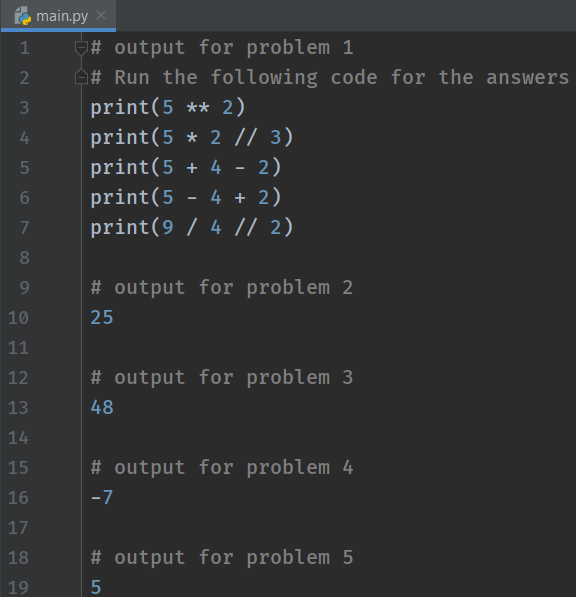## What Next?

To conclude, you are firmly on your way to become a successful Python developer!

Subsequently, in our next tutorial, we will discuss an important topic called Variables. A variable helps us store a value. Moreover, variables store the expressions that we have evaluated in this tutorial. Therefore, let's head to the next tutorial.

Happy Coding :)